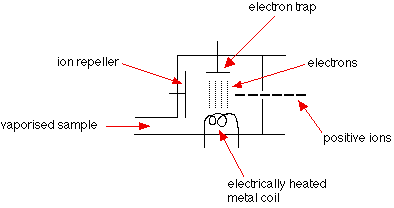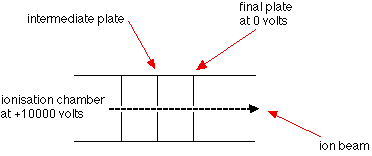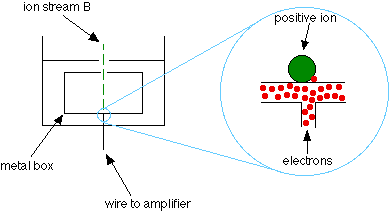THE MASS SPECTROMETER

```
```

This page describes how a mass spectrum is produced using a mass spectrometer. In fact, there are several different designs of mass spectrometer which differ in detail - this page looks at one which is easy to understand.

```
```

How a mass spectrometer works

The basic principle

If something is moving and you subject it to a sideways force, instead of moving in a straight line, it will move in a curve - deflected out of its original path by the sideways force.

Suppose you had a cannonball travelling past you and you wanted to deflect it as it went by you. All you've got is a jet of water from a hose-pipe that you can squirt at it. Frankly, its not going to make a lot of difference! Because the cannonball is so heavy, it will hardly be deflected at all from its original course.

But suppose instead, you tried to deflect a table tennis ball travelling at the same speed as the cannonball using the same jet of water. Because this ball is so light, you will get a huge deflection.

The amount of deflection you will get for a given sideways force depends on the mass of the ball. If you knew the speed of the ball and the size of the force, you could calculate the mass of the ball if you knew what sort of curved path it was deflected through. The less the deflection, the heavier the ball.

Note:  I'm not suggesting that you personally would have to do the calculation, although the maths isn't actually very difficult - certainly no more than A'level standard!

You can apply exactly the same principle to atomic sized particles.

```
```

An outline of what happens in a mass spectrometer

Atoms and molecules can be deflected by magnetic fields - provided the atom or molecule is first turned into an ion. Electrically charged particles are affected by a magnetic field although electrically neutral ones aren't.

The sequence is :

Stage 1: Ionisation

The atom or molecule is ionised by knocking one or more electrons off to give a positive ion. This is true even for things which you would normally expect to form negative ions (chlorine, for example) or never form ions at all (argon, for example). Most mass spectrometers work with positive ions.

Note:  All mass spectrometers that you will come across if you are doing a course for 16 - 18 year olds work with positive ions. Even if a few atoms in a sample of chlorine, for example, captured an electron instead of losing one, the negative ions formed wouldn't get all the way through the ordinary mass spectrometer. But it has been pointed out to me that there is work being done on negative ion mass spectrometers, although they use a different ionisation technique.

My thanks to Professor John Todd of the University of Kent for drawing this to my attention.

Stage 2: Acceleration

The ions are accelerated so that they all have the same kinetic energy.

Stage 3: Deflection

The ions are then deflected by a magnetic field according to their masses. The lighter they are, the more they are deflected.

The amount of deflection also depends on the number of positive charges on the ion - in other words, on how many electrons were knocked off in the first stage. The more the ion is charged, the more it gets deflected.

Stage 4: Detection

The beam of ions passing through the machine is detected electrically.

```
```

A full diagram of a mass spectrometer```
```

Understanding what's going on

The need for a vacuum

It's important that the ions produced in the ionisation chamber have a free run through the machine without hitting air molecules.

IonisationThe vaporised sample passes into the ionisation chamber. The electrically heated metal coil gives off electrons which are attracted to the electron trap which is a positively charged plate.

The particles in the sample (atoms or molecules) are therefore bombarded with a stream of electrons, and some of the collisions are energetic enough to knock one or more electrons out of the sample particles to make positive ions.

Most of the positive ions formed will carry a charge of +1 because it is much more difficult to remove further electrons from an already positive ion.

These positive ions are persuaded out into the rest of the machine by the ion repeller which is another metal plate carrying a slight positive charge.

Note:  As you will see in a moment, the whole ionisation chamber is held at a positive voltage of about 10,000 volts. Where we are talking about the two plates having positive charges, these charges are in addition to that 10,000 volts.

AccelerationThe positive ions are repelled away from the very positive ionisation chamber and pass through three slits, the final one of which is at 0 volts. The middle slit carries some intermediate voltage. All the ions are accelerated into a finely focused beam.

DeflectionDifferent ions are deflected by the magnetic field by different amounts. The amount of deflection depends on:

• the mass of the ion. Lighter ions are deflected more than heavier ones.

• the charge on the ion. Ions with 2 (or more) positive charges are deflected more than ones with only 1 positive charge.

These two factors are combined into the mass/charge ratio. Mass/charge ratio is given the symbol m/z (or sometimes m/e).

For example, if an ion had a mass of 28 and a charge of 1+, its mass/charge ratio would be 28. An ion with a mass of 56 and a charge of 2+ would also have a mass/charge ratio of 28.

In the last diagram, ion stream A is most deflected - it will contain ions with the smallest mass/charge ratio. Ion stream C is the least deflected - it contains ions with the greatest mass/charge ratio.

It makes it simpler to talk about this if we assume that the charge on all the ions is 1+. Most of the ions passing through the mass spectrometer will have a charge of 1+, so that the mass/charge ratio will be the same as the mass of the ion.

Note:  You must be aware of the possibility of 2+ (etc) ions, but the vast majority of A'level questions will give you mass spectra which only involve 1+ ions. Unless there is some hint in the question, you can reasonably assume that the ions you are talking about will have a charge of 1+.

Assuming 1+ ions, stream A has the lightest ions, stream B the next lightest and stream C the heaviest. Lighter ions are going to be more deflected than heavy ones.

Detection

Only ion stream B makes it right through the machine to the ion detector. The other ions collide with the walls where they will pick up electrons and be neutralised. Eventually, they get removed from the mass spectrometer by the vacuum pump.When an ion hits the metal box, its charge is neutralised by an electron jumping from the metal on to the ion (right hand diagram). That leaves a space amongst the electrons in the metal, and the electrons in the wire shuffle along to fill it.

A flow of electrons in the wire is detected as an electric current which can be amplified and recorded. The more ions arriving, the greater the current.

Detecting the other ions

How might the other ions be detected - those in streams A and C which have been lost in the machine?

Remember that stream A was most deflected - it has the smallest value of m/z (the lightest ions if the charge is 1+). To bring them on to the detector, you would need to deflect them less - by using a smaller magnetic field (a smaller sideways force).

To bring those with a larger m/z value (the heavier ions if the charge is +1) on to the detector you would have to deflect them more by using a larger magnetic field.

If you vary the magnetic field, you can bring each ion stream in turn on to the detector to produce a current which is proportional to the number of ions arriving. The mass of each ion being detected is related to the size of the magnetic field used to bring it on to the detector. The machine can be calibrated to record current (which is a measure of the number of ions) against m/z directly. The mass is measured on the 12C scale.

Note:  The 12C scale is a scale on which the 12C isotope weighs exactly 12 units.

```
```

What the mass spectrometer output looks like

The output from the chart recorder is usually simplified into a "stick diagram". This shows the relative current produced by ions of varying mass/charge ratio.

The stick diagram for molybdenum looks lilke this:You may find diagrams in which the vertical axis is labelled as either "relative abundance" or "relative intensity". Whichever is used, it means the same thing. The vertical scale is related to the current received by the chart recorder - and so to the number of ions arriving at the detector: the greater the current, the more abundant the ion.

As you will see from the diagram, the commonest ion has a mass/charge ratio of 98. Other ions have mass/charge ratios of 92, 94, 95, 96, 97 and 100.

That means that molybdenum consists of 7 different isotopes. Assuming that the ions all have a charge of 1+, that means that the masses of the 7 isotopes on the carbon-12 scale are 92, 94, 95, 96, 97, 98 and 100.

Note:  If there were also 2+ ions present, you would know because every one of the lines in the stick diagram would have another line at exactly half its m/z value (because, for example, 98/2 = 49). Those lines would be much less tall than the 1+ ion lines because the chances of forming 2+ ions are much less than forming 1+ ions.

If you want to go straight on to how you use these mass spectra to calculate relative atomic masses you can jump straight to that page by following this link rather than going via the menus below.

```
```
 Questions to test your understanding If this is the first set of questions you have done, please read the introductory page before you start. You will need to use the BACK BUTTON on your browser to come back here afterwards. questions on how a mass spectrometer works answers
```
```

Where would you like to go now?

To the mass spectrometry menu . . .

To the instrumental analysis menu . . .

To Main Menu . . .

```
```